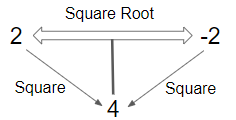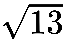# Square Root Formula

## Square Root Formulas with Examples

The square root of a number is another number which produces the first number when it is multiplied by itself.
For example,
The square root of 4 is 2.

A square root is written with a radical symbol $\sqrt {}$and the number or expression inside the radical symbol is called the radicand.
e.g. $\sqrt 4$, here 4 is the radicand.

All positive real numbers has two square roots, one positive square root and one negative square root. The positive square root is sometimes referred as principal square root.
e.g. $\sqrt 4 = \pm 2$

The product of two numbers is positive if both numbers have the same sign as it the case with squares and square roots.For e.g. 4 = 2 . 2 = (-2) . (-2)

To calculate the square root, first of all we should try to find out the factors of that number. Then we have to group the pairs of factors. If we can’t find the factors of that number i.e. its an irrational number. Then the square root of that number will be in decimal form which won’t terminate or repeat.

e.g. $\sqrt {12} = \sqrt {4 \times 3} = 2\sqrt 3$
irrational number: $\sqrt {17} \approx 4.1231....$

Solved Examples

Q.1.What is the square root of 81?

Solution: The factors of 81 are given as,
81 = 9 × 9
$\therefore \sqrt {81} = \sqrt {9 \times 9} = 9$
Hence, the square root of 81 is 9.

Q.2. What is the square root of 112 ?

Solution: The factors of 112 are given as,
$\begin{gathered} 112 = 2 \times 2 \times 2 \times 2 \times 7 \hfill \\ = (2 \times 2) \times (2 \times 2) \times 7 \hfill \\ \therefore \sqrt {112} = \sqrt {(2 \times 2) \times (2 \times 2) \times 7} \hfill \\ \therefore \sqrt {112} = 2 \times 2\sqrt 7 \hfill \\ \therefore \sqrt {112} = 4\sqrt 7 \hfill \\ \end{gathered}$

Hence the square root of 112 is $4\sqrt 7$

Practise Question:What is the square root of 117?
Option (a) : 3Option (b) : 5Option (c) : 7Option (d) : 9